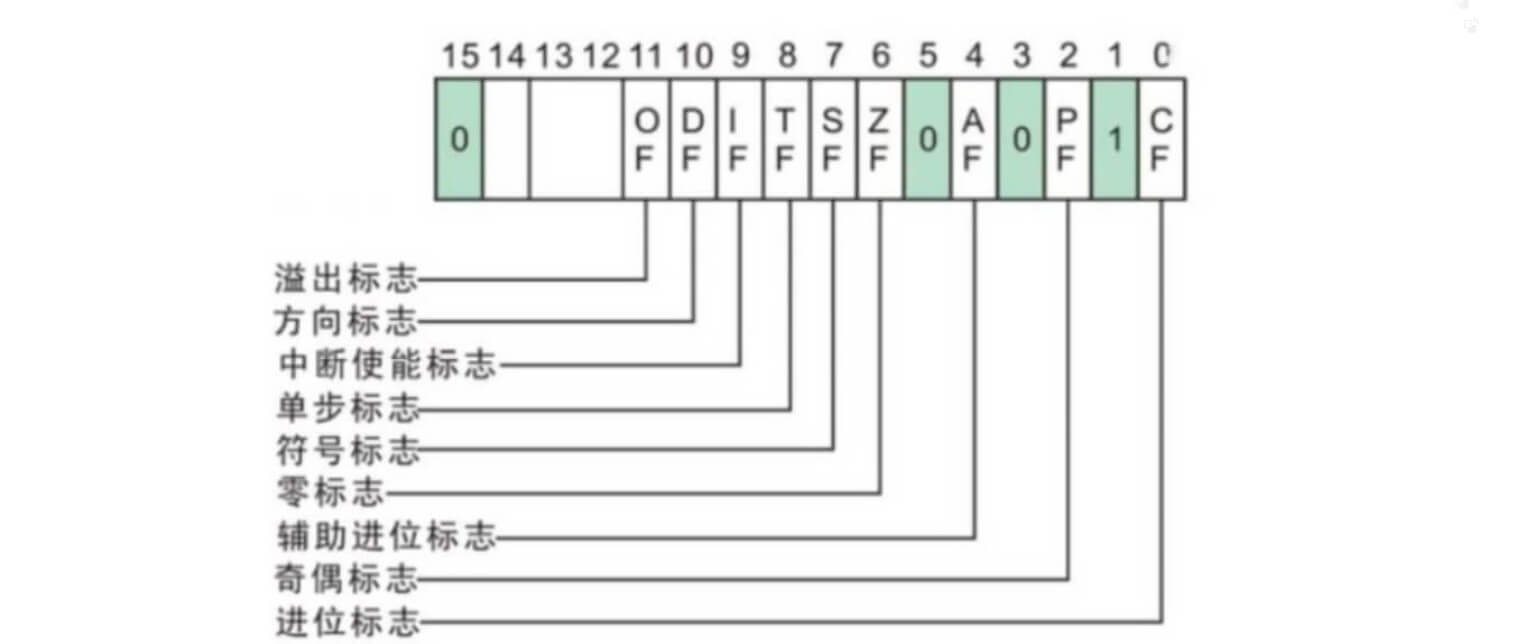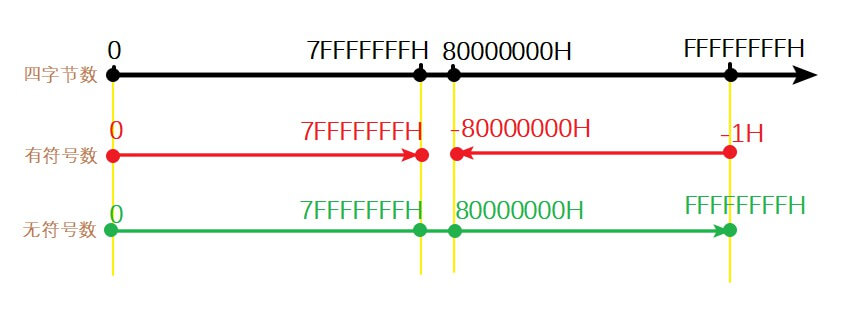# 标志位

## 奇偶标志P

• ADD 8930, 0的结果显示P=1。8930H的二进制为1000 1001 0011 0000B，这个数中最低字节有两个1，总共五个1
• ADD 8931, 0的结果显示P=0。尽管总共六个1，但是最低字节有3个1

## 符号标志S

• 如果是无符号数：四字节数的表面值就等于实际值；
• 如果是有符号数：在有限的取值范围下，为了保证正数和负数数目相同，规定 00 00 00 00 ~ 7F FF FF FF 表示相当于十进制的 0 ~ 21,4748,3647，而 80 00 00 00 ~ FF FF FF FF 表示相当于十进制中的 -21,4748,3648 ~ -1。# 跳转指令

• 检查标志位进行跳转
• 检查两个操作数进行跳转
• 比较两个无符号数进行跳转
• 比较两个有符号数进行跳转

## 基于标志位

Description Flag J- 跳转1 JN- 跳转0

## 比较两个操作数

### 比较两个普通操作数

JEJNE根据标志位Z的结果判定是否需要跳转。JE和JZ、JNE和JNE实际上是等效的，都是以标志位Z为依据进行跳转。它们的不同在于直观理解上：

• 后缀E（Equal）倾向于判断两个操作数是否相同
• 后缀Z（Zero）倾向于检查标志位Z的值

### 检查寄存器值是否为0

• JECXZ 表示当ECX=0时跳转
• JCXZ 表示当CX=0时跳转

## 比较两个无符号数

$\text{left} < \text{right}$ 小于 JB 不大于等于 JNAE
$\text{left} \le \text{right}$ 小于等于 JBE 不大于 JNA
$\text{left} = \text{right}$ 等于 JE 既不大于也不小于 JNAB （这个指令不存在）
$\text{left} > \text{right}$ 大于 JA 不小于等于 JNBE
$\text{left} \ge \text{right}$ 大于等于 JAE 不小于 JNB

## 比较两个有符号数

$\text{left} < \text{right}$ 小于 JL 不大于等于 JNGE
$\text{left} \le \text{right}$ 小于等于 JLE 不大于 JNG
$\text{left} = \text{right}$ 等于 JE 既不大于也不小于 JNGL （这个指令不存在）
$\text{left} > \text{right}$ 大于 JG 不小于等于 JNLE
$\text{left} \ge \text{right}$ 大于等于 JGE 不小于 JNL
>>>>>>>>>>>>> 转载请注明出处 <<<<<<<<<<<<<
0%